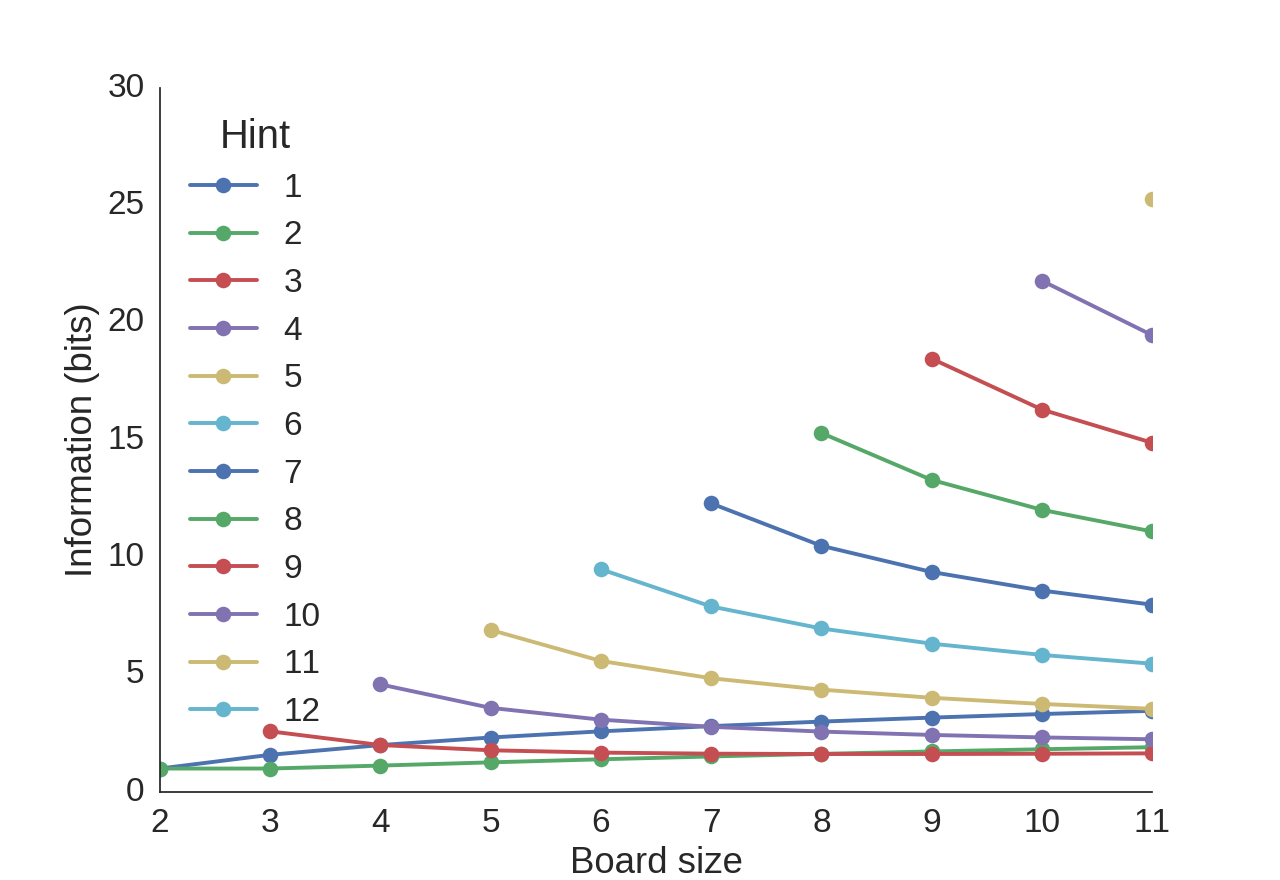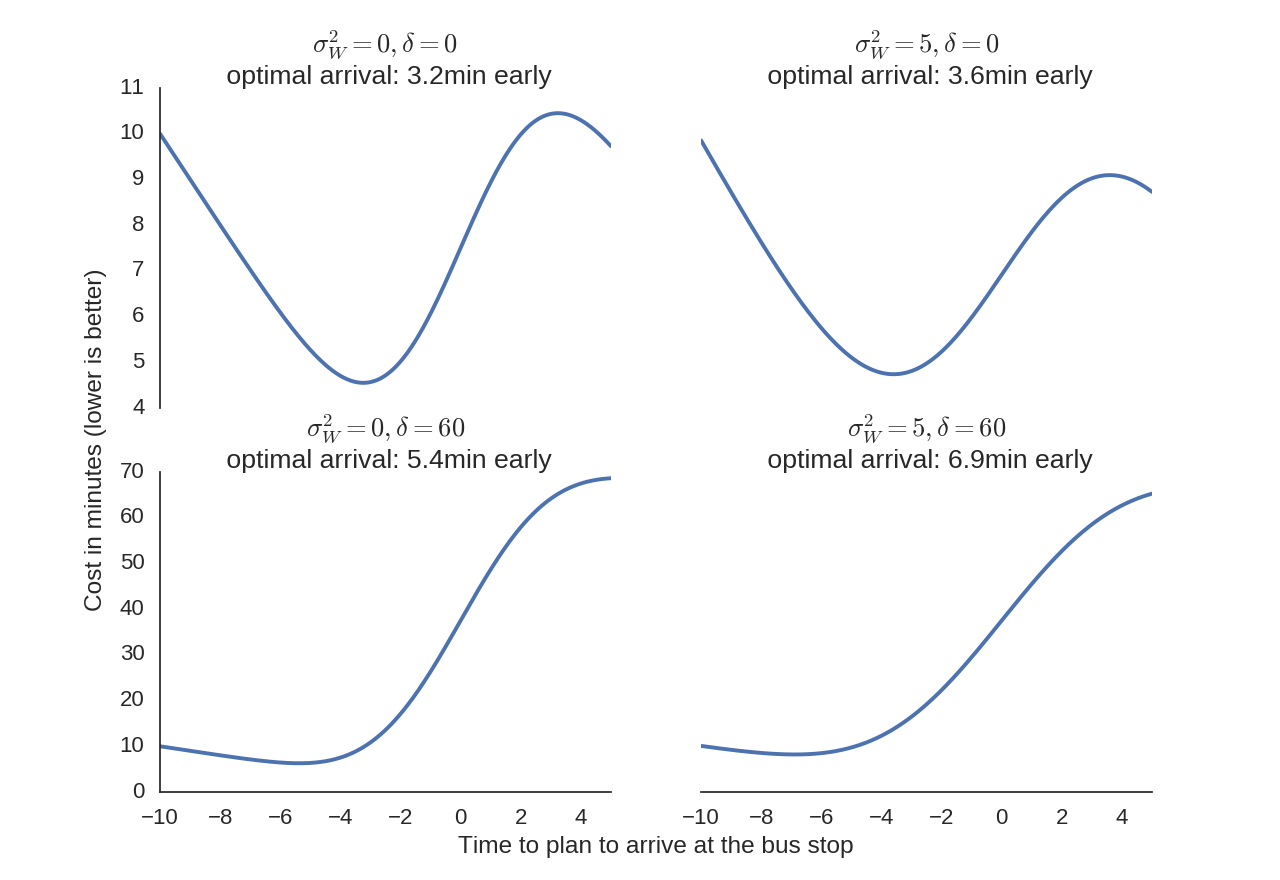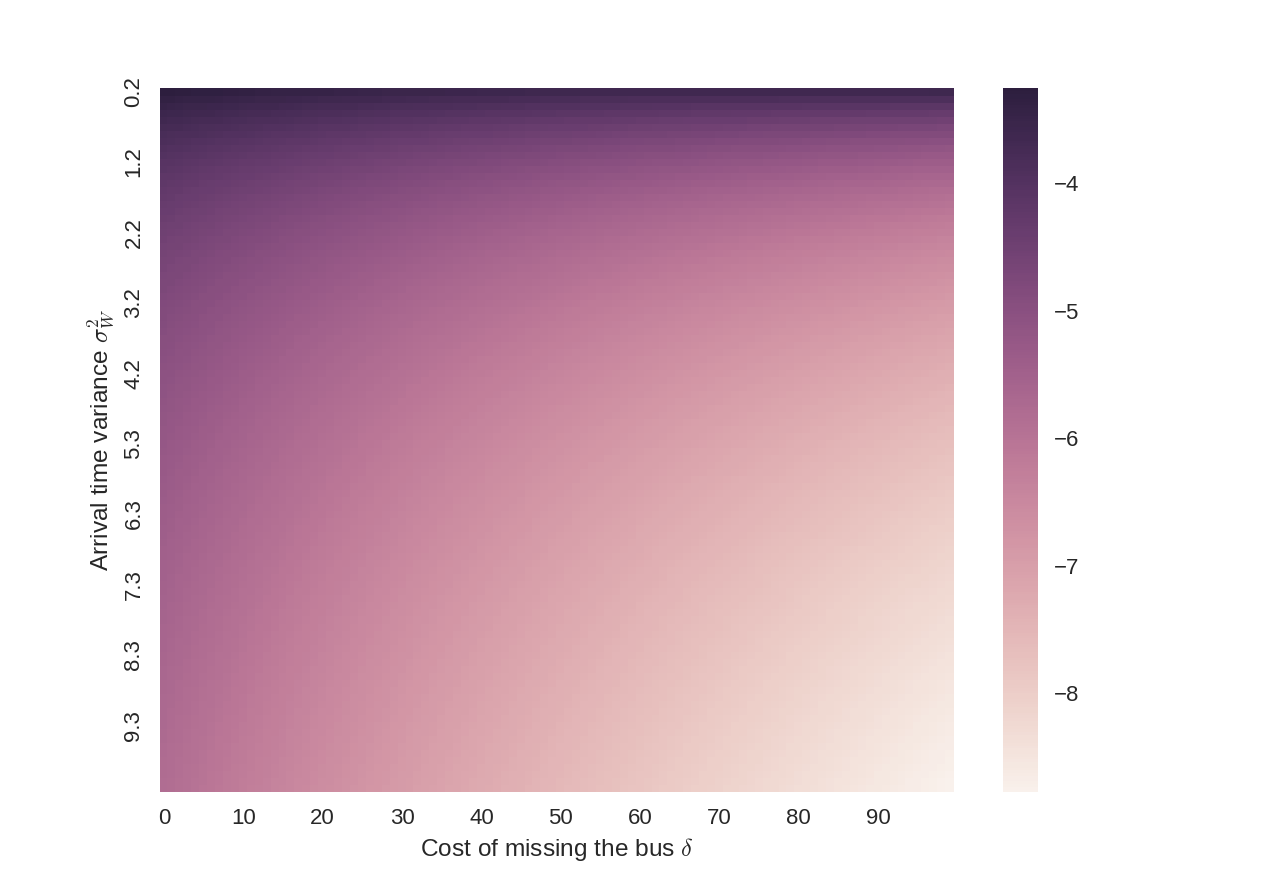There is a wonderful collection of puzzles by Simon Tatham called the Portable Puzzle Collection which serves as a fun distraction. The game “Towers” is a simple puzzle where you must fill in a Latin square with numbers $$1 \ldots N$$, only one of each per row/column, as if the squares contained towers of this height. The number of towers visible from the edges of rows and columns are given as clues. For example,

Solved, the board would appear as,

In more advanced levels, not all of the hints are given. Additionally, in these levels, hints can also be given in the form of the value of particular cells. For example, the initial conditions of the puzzle may be,

With such different types of hints, it raises the question of whether some hints are better than others.

## How will we approach the problem?

We will use an information-theoretic framework to understand how useful different hints are. This allows us to measure the amount of information that a particular hint gives about the solution to a puzzle in bits, a nearly-identical unit to that used by computers to measure file and memory size.

Information theory is based on the idea that random variables (quantities which can take on one of many values probabilistically) are not always independent, so sometimes knowledge of the value of one random variable can change the probabilities for a different random variable. For instance, one random variable may be a number 1-10, and a second random variable may be whether that number is even or odd. A bit is an amount of information equal to the best possible yes or no question, or (roughly speaking) information that can cut the number of possible outcomes in half. Knowing whether a number is even or odd gives us one bit of information, since it specifies that the first random variable can only be one of five numbers instead of one of ten.

Here, we will define a few random variables. Most importantly, we will have the random variable describing the correct solution of the board, which could be any possible board. We will also have random variables which represent hints. There are two types of hints: initial cell value hints (where one of the cells is already filled in) and tower visibility hints (which define how many towers are visible down a row or column).

The number of potential Latin squares of size $$N$$ grows very fast. For a $$5×5$$ square, there are 161,280 possibilities, and for a $$10×10$$, there are over $$10^{47}$$. Thus, for computational simplicity, we analyze a $$4×4$$ puzzle with a mere 576 possibilities.

## How useful are “initial cell value” hints?

First, we measure the entropy, or the maximal information content that a single cell will give. For the first cell chosen, there is an equal probability that any of the values (1, 2, 3, or 4) will be found in that cell. Since there are two options, this give us 2 bits of information.

What about the second initial cell value? Interestingly, it depends both on the location and on the value. If the second clue is in the same row or column as the first, it will give less information. If it is the same number as the first, it will also give less information.

Counter-intuitively, in the 4×4 board, this means we gain more than 2 bits of information from the second hint. This is because, once we reveal the first cell’s value, the probabilities of each of the other cell’s possible values are not equal as they were before. Since we are not choosing from the same row or column of our first choice, is more likely that this cell will be equal to the first cell’s value than to any other value. So therefore if we reveal a value which is different, it will provide more information.

Intuitively, for the 4×4 board, suppose we reveal the value of a cell and it is 4. There cannot be another 4 in the same column or row, so if we are to choose a hint from a different column or row, we are effectively choosing from a leaving a 3×3 grid. There must be 3 4 values in the 3×3 grid, so the probability of selecting it is 1/3. We have an even probability of selecting a 1, 2, or 3, so each other symbol has a probability of 2/9. Being more surprising finds, we gain 2.17 bits of information from each of these three.

Consequently, selecting a cell in the same row or column, or one which has the same value as the first, will give an additional 1.58 bits of information.

## How about “tower visibility” hints?

In a 4×4 puzzle, it is very easy to compute the information gained if the hint is a 1 or a 4. A hint of 1 always gives the same amount of information as a single square: it tells us that the cell on the edge of the hint must be a 4, and gives no information about the rest of the squares. If only one tower can be seen, the tallest tower must come first. Thus, it must give 2 bits of information.

Additionally, we know that if the hint is equal to 4, the only possible combination for the row is 1, 2, 3, 4. Thus, this gives an amount of information equal to the entropy of a single row, which turns out to be 4.58 bits.

For a hint of 2 or 3, the information content is not as immediately clear, but we can calculate them numerically. For a hint of 2, we have 1.13 bits, and for a hint of 3, we have 2 bits.

Conveniently, due to the fact that the reduction of entropy in a row must be equal to the reduction of entropy in the entire puzzle, we can compute values for larger boards. Below, we show the information gained about the solution from each possible hint (indicated by the color). In general, it seems higher hints are usually better, but a hint of 1 is generally better than one of 2 or 3.For each board size, the information content of each potential hint is plotted.

## Conclusion

In summary:

• The more information given by a hint for a puzzle, the easier that hint makes it to solve the puzzle.
• Of the two types of hints, usually the hints about the tower visibility are best.
• On small boards (of size less than 5), hints about individual cells are very useful.
• The more towers visible from a row or column, the more information is gained about the puzzle from that hint.

Of course, remember that all of the hints combined of any given puzzle must be sufficient to completely solve the puzzle (assuming the puzzle is solvable), so the information content provided by the hints must be equal to the entropy of the puzzle of the given size. When combined, we saw in the “initial cell value” that hints may become more or less effective, so these entropy values cannot be directly added to determine which hints provide the most information. Nevertheless, this serves as a good starting point in determining which hints are the most useful.

### Theoretical note

For initial cell hints, it is possible to compute the information content analytically for any size board. For a board of size $$N×N$$ with $$N$$ symbols, we know that the information contained in the first hint is $$-\log(1/N)$$ bits. Suppose this play uncovers token X. Using this first play, we construct a sub-board where the row and column of the first hint are removed, leaving us with an $$(N-1)×(N-1)$$ board. If we choose a cell from this board, it has a $$1/(N-1)$$ probability of being X and an equal chance of being anything else, giving a $$\frac{N-2}{(N-1)^2}$$ probability of each of the other tokens. Thus, information gained is $$-\frac{N-2}{(N-1)^2}×\log\left(\frac{N-2}{(N-1)^2}\right)$$ if the value is different from the first, and $$-1/(N-1)×\log\left(1/(N-1)\right)$$ if they are the same; these expressions are approximately equal for large $$N$$. Note how no information is gained when the second square is revealed if $$N=2$$.

Similarly, when a single row is revealed (for example by knowing that $$N$$ towers are visible from the end of a row or column) we know that the entropy must be reduced by $$-\sum_{i=1}^N \log(1/N)$$. This is because the first element revealed in the row gives $$-\log(1/N)$$ bits, the second gives $$-\log(1/(N-1))$$ bits, and so on.

### Solving a puzzle algorithmically

Most of these puzzles are solvable without backtracking, i.e. the next move can always be logically deduced from the state of the board without the need for trial and error. By incorporating the information from each hint into the column and row states and then integrating this information across rows and columns, it turned out to be surprisingly simple to write a quick and dirty algorithm to solve the puzzles. This algorithm, while probably not of optimal computational complexity, worked reasonably well. Briefly,

1. Represent the initial state of the board by a length-$$N$$ list of lists, where each of the $$N$$ lists represents a row of the board, and each sub-list contains all of the possible combinations of this row (there are $$N!$$ of them to start). Similarly, define an equivalent (yet redundant) data structure for the columns.
2. Enforce each condition on the start of the board by eliminating the impossible combinations using the number of towers visible from each row and column, and using the cells given at initialization. Update the row and column lists accordingly.
3. Now, the possible moves for certain squares will be restricted by the row and column limitations; for instance, if only 1 tower is visible in a row or column, the tallest tower in the row or column must be on the edge of the board. Iterate through the cells, restricting the potential rows by the limitations on the column and vice versa. For example, if we know the position of the tallest tower in a particular column, eliminate the corresponding rows which do not have the tallest tower in this position in the row.
4. After sufficient iterations of (3), there should only be one possible ordering for each row (assuming it is solvable without backtracking). The puzzle is now solved.

This is not a very efficient algorithm, but it is fast enough and memory-efficient enough for all puzzles which might be fun for a human to solve. This algorithm also does not work with puzzles which require backtracking, but could be easily modified to do so.

### Code/Data:

Anyone who has taken the bus has at one time or another wondered, “When should I plan to be at the bus stop?” or more importantly, “When should I leave if I want to catch the bus?” Many bus companies suggest arriving a few minutes early, but there seem to be no good analyses on when to leave for the bus. I decided to find out.

## Finding a cost function

Suppose we have a bus route where a bus runs every $$I$$ minutes, so if you don’t catch your bus, you can always wait for the next bus. However, since more than just your time is at stake for missing the bus (e.g. missed meetings, stress, etc.), we assume there is a penalty $$\delta$$ for missing the bus in addition to the extra wait time. $$\delta$$ here is measured in minutes, i.e. how many minutes of your time would you exchange to be guaranteed to avoid missing the bus. $$\delta=0$$ therefore means that you have no reason to prefer one bus over another, and that you only care about minimizing your lifetime bus wait time.

Assuming we will not be late enough to need to catch the third bus, we can model this with two terms, representing the cost to you (in minutes) of catching each of the next two buses, weighted by the probability that you will catch that bus:

$C(t) = \left(E(T_B) - t\right) P\left(T_B > t + L_W\right) + \left(I + E(T_B) - t + \delta\right) P(T_B < t + L_W)$

where $$T_B$$ is the random variable representing the time at which the bus arrives, $$L_W$$ is the random variable respresenting the amount of time it takes to walk to the bus stop, and $$t$$ is the time you leave. ($$E$$ is expected value and $$P$$ is the probability.) We wish to choose a time to leave the office $$t$$ which minimizes the cost function $$C$$.

If we assume that $$T_B$$ and $$L_W$$ are Gaussian, then it can shown that the optimal time to leave (which minimizes the above function) is

$t = -\mu_W - \sqrt{\left(\sigma_B^2 + \sigma_W^2\right)\left(2\ln\left(\frac{I+\delta}{\sqrt{\sigma_B^2+\sigma_W^2}}\right)-2\ln\left(\sqrt{2\pi}\right)\right)}$

where $$\sigma_B^2$$ is the variance of the bus arrival time, $$\sigma_W^2$$ is the variance of your walk, and $$\mu_W$$ is expected duration of your walk. In other words, you should plan to arrive at the bus stop on average $$\sqrt{\left(\sigma_B^2 + \sigma_W^2\right)\left(2\ln\left(\left(I+\delta\right)/\sqrt{\sigma_B^2+\sigma_W^2}\right)-2\ln\left(\sqrt{2\pi}\right)\right)}$$ minutes before your bus arrives.

Note that one deliberate oddity of the model is that the cost function does not just measure wait time, but also walking time. I optimized on this because, in the end, what matters is the total time you spend getting on the bus.

## What does this mean?

The most important factor you should consider when choosing which bus to take is the variability in the bus’ arrival time and the variability in the time it takes you to walk to the bus. The arrival time scales approximately linearly with the standard deviation of the variability.

Additionally, it scales at approximately the square root of the log the your value of time and of the frequency of the buses. So even if very high values of time and very infrequent buses do not substantially change the time at which you should arrive. For approximation purposes, you might consider adding a constant in place of this term, anywhere from 2-5 minutes depending on the frequency of the bus.

## Checking the assumption

First, we need to collect some data to assess whether the bus time arrival ($$T_B$$) is normally distributed. I wrote scripts to scrape data from Yale University’s Blue Line campus shuttle route. Many bus systems (including Yale’s) now have real-time predictions, so I used many individual predictions by Yale’s real-time arrival prediction system as the expected arrival time, simulating somebody checking this to see when the next bus comes.

For our purposes, the expected arrival time looks close enough to a Gaussian distribution:

## So what time should I leave?

When estimating the $$\sigma_B^2$$ parameter, we only examine bus times which are 10 minutes away or later. This is because you can’t use a real-time bus system to plan ahead of time to catch something if it is too near in the future, which defeats the purpose of the present analysis. The variance in arrival time for the Yale buses is $$\sigma_B^2=5.7$$.

We use an inter-bus interval of $$I=15$$ minutes.

While the variability of the walk to the bus station $$\sigma_W^2$$ is unique for each person, I consider two cases: one case, where we assume that arrival time variability is small ($$\sigma_W^2=0$$) compared to the bus’ variability, representing the case where the bus stop is (for intance) located right outside one’s office building. I also consider the case where the time variability is comperable to the variability for the bus ($$\sigma_W=5$$), representing the case where one must walk a long distance to the bus stop.

Finally, I consider the case where we strongly prioritize catching the desired bus ($$\delta=60$$ corresponding to, e.g., an important meeting) and also the case where we seek to directly minimize the expected wait time ($$\delta=0$$ corresponding to, e.g., the commute home).Even though the shape of the optimization function changes greatly, the optimal arrival time changes very little.

We can also look at a spectrum of different cost tradeoffs for missing the bus (values of $$\delta$$) and variance in the walk time (values of $$\sigma_W^2 = var(W)$$). Because they appear similarly in the equations, we can also consider these values to be changes in the interval of the bus arrival $$I$$ and the variance of the bus’ arrival time $$\sigma_B^2=var(B)$$ respectively.Across all reasonable values, the optimal time to plan to arrive is between 3.5 and 8 minutes early.

## Conclusion

So to summarize:

• If it always takes you approximately the same amount of time to walk to the bus stop, plan to be there 3-4 minutes early on your commute home, or 5-6 minutes early if it’s the last bus before an important meeting.
• If you have a long walk to the bus stop which can vary in duration, plan to arrive at the bus stop 4-5 minutes early if you take the bus every day, or around 7-8 minutes early if you need to be somewhere for a special event.
• These estimations assume that you know how long it takes you on average to walk to the bus stop. As we saw previously, if you need to be somewhere at a certain time, arriving a minute early is much better than arriving a minute late. If you don’t need to be somewhere, just make your best guess.
• The best way to reduce waiting time is to decrease variability.
• These estimates also assume that the interval between buses is sufficiently large. If it is small, as in the case of a subway, there are different factors that govern the time you spend waiting.
• This analysis focuses on buses with an expected arrival time, not with a scheduled arrival time. When buses have schedules, they will usually wait at the stop if they arrive early. This situation would require a different analysis than what was performed here.